# Part 8 of the 4D basics: Wirtinger derivatives.

This is the 91-th post on this website so surely but slowly this website is growing on. This post was more or less written just for myself; I don’t know if the concept of Wirtinger derivates is used a lot in standard complex analysis but I sure like it so that’s why we take a look at it.

The idea of a Wirtinger derivative is very simple to understand: You have some function f(Z) and by differentiating it in the direction of all four basis vectors you craft the derivative f'(Z) from that.

At the basis for all the calculations we do in this post are the Cauchy-Riemann equations that allow you to rewrite the partial derivatives we put into the Wirtinger derivative.

The main result in this post is as follows:
We take our Wirtinger operator W and we multiply it with the 4D complex conjugate of W and we show that this is a real multiple of the Laplacian.

The 4D case is more or less the same as on the complex plane, that is not a miracle because in previous 4D basics we already observed two planes inside the 4D complex numbers that are isomorphic to the complex plane. So it is not much of a surprise the entire 4D space of complex numbers behaves in that way too; all functions are harmonic that is the Laplacian of such a function is zero.

This post is ten pictures long, most of them are size 550 x 775 but a few of them are a bit broader like 600 x 775 because the calculations are rather wide.

On the scale of things this post is not ultra important or so, it is more like I wrote it for myself and I wanted to look in how much this all was different from the three dimensional case.

Here are the pics: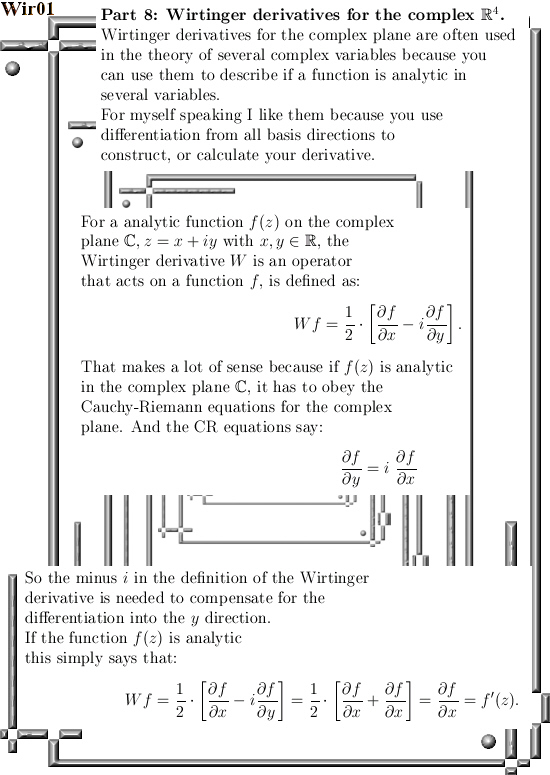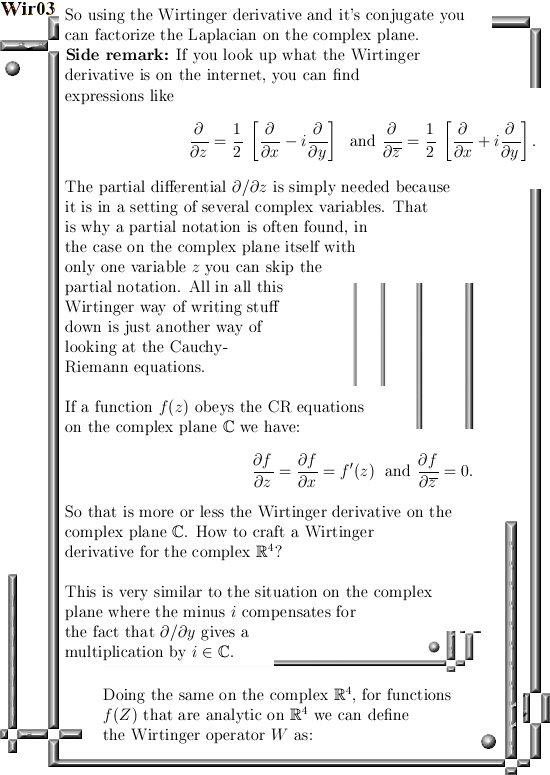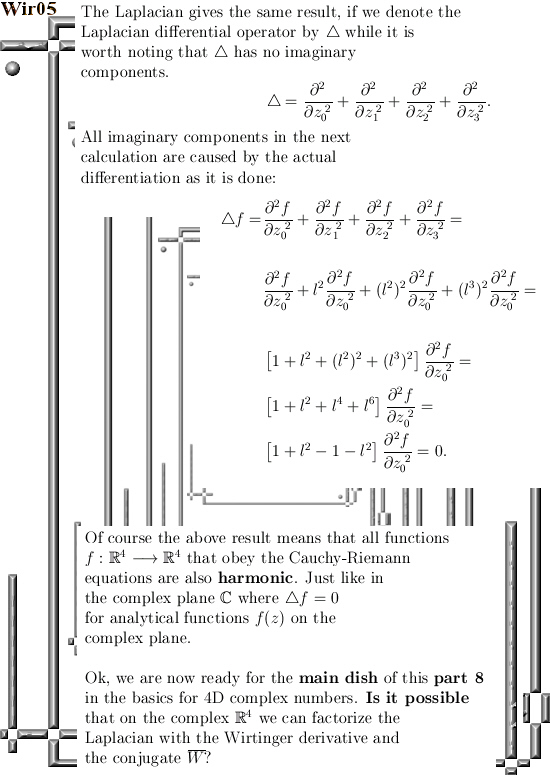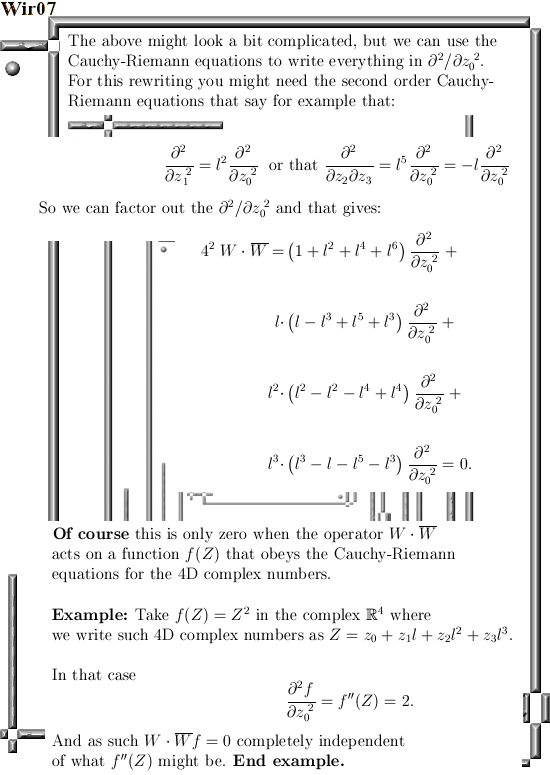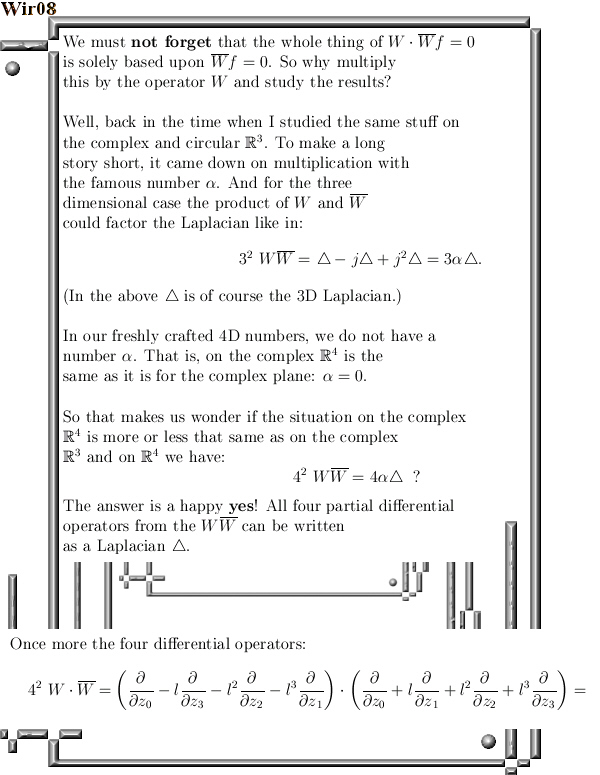Further reading from a wiki (of course that is only about 2D complex numbers from the complex plane):
Wirtinger derivatives
https://en.wikipedia.org/wiki/Wirtinger_derivatives

Ok, that was it. Till updates.

# Wirtinger derivatives and the factorization of the Laplacian.

This post could have many titles, for example ‘Factorization of the Laplacian using second order Cauchy-Riemann equations’ would also cover what we will read in the next seven pictures.

The calculation as shown below is, as far as I am concerned, definitely in the top ten of results relating to all things 3D complex numbers. Only when I stumbled on this a few years back I finally understood the importance of the so called sphere-cone equation.

The calculation below is basically what you do when writing out the sphere-cone equation only now it is not with variables like x, y and z but with the partial differential operators with respect to x, y and z. In simplifying the expressions we get I use so called second order Cauchy-Riemann equations, if you understand the standard CR equations these second order equations are relatively easy to digest.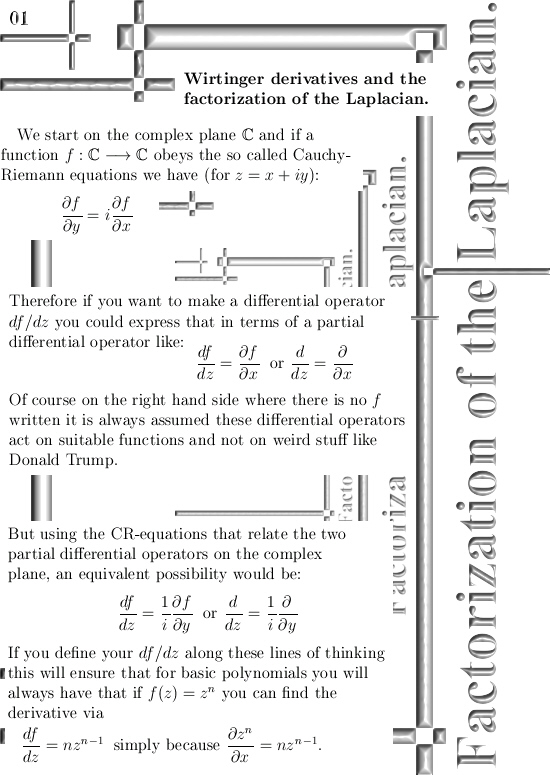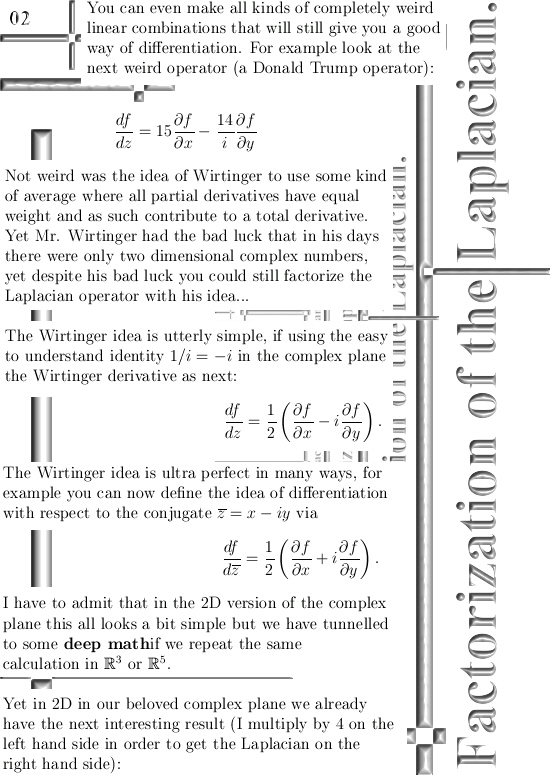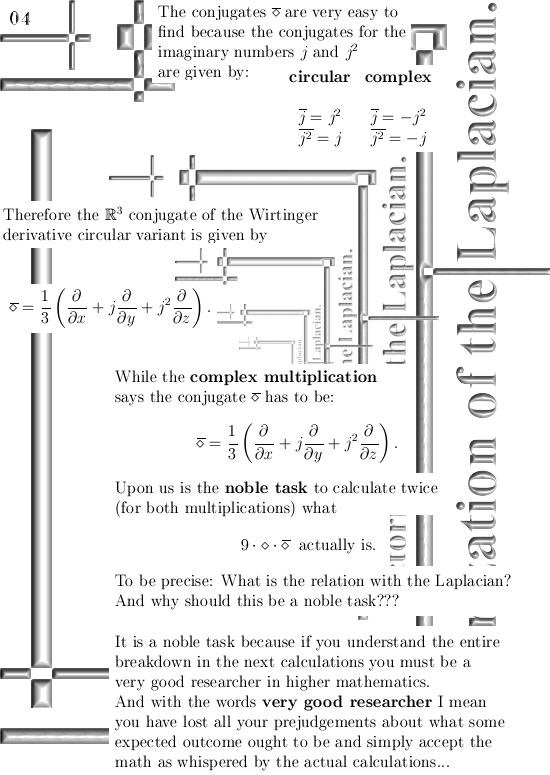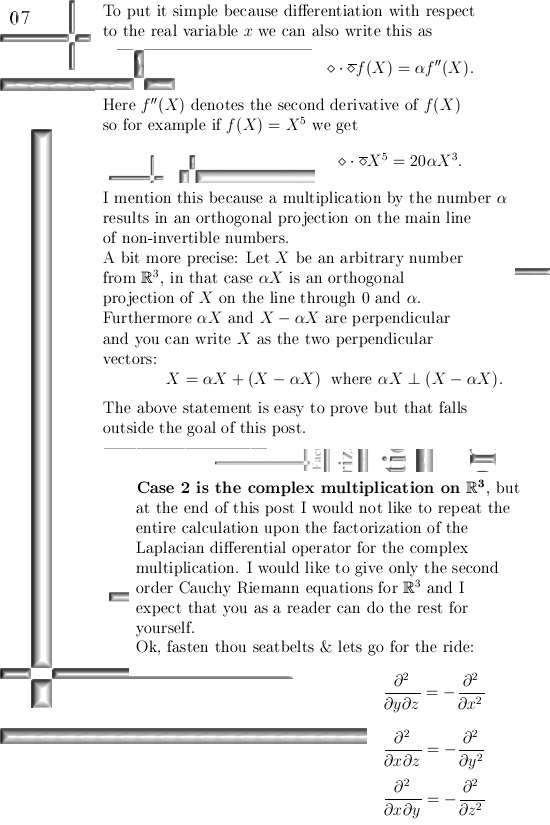This post is also categorized under Quantum Mechanics, the reason for that is that the wave equation contains the Laplacian operator and the more you know about that rather abstract thing the better it is in my view.

I would like to close with a link to a wiki on Wirtinger derivatives, originally they come from theory with several complex variables. That explains why in the wiki the Wirtinger derivatives are written as partial derivatives while above we can use the straight d´s for our differential of f.

Here is the wiki: Wirtinger derivatives
https://en.wikipedia.org/wiki/Wirtinger_derivatives

# Schrödinger wave equation part 2.

A few posts back I wrote a bit about the Schrödinger wave equation related to calculating atomic and molecular orbitals for electrons using 3D complex numbers.

What I said was basically correct but also an over-simplification of the situation.
The problem is very very basic: in the 3D number system, let it be complex or circular, you just cannot solve and equation like \$X^2 = -1\$.
Hence the number i from the complex plane with i^2 = -1 just does not live in 3D real space.

So using alternative number systems outside the complex plane is not a straightforward thing to do, yet in principle all higher dimensional complex numbers should give the same results.
If not there would be a very basic problem inside the wave equation from quantum mechanics and I am not aware of any faults in that detail of the quantum theory.

Here are two pictures that serve as an addendum on the previous post on the Schrödinger equation: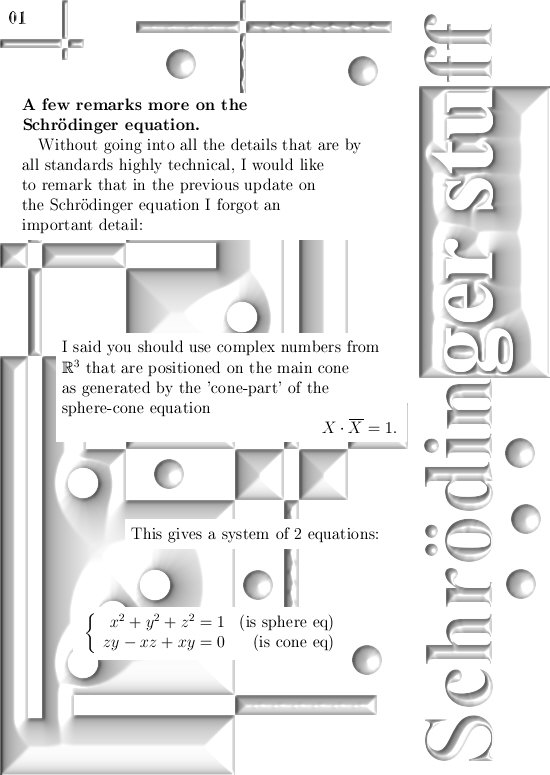Now if you are reading this it is very likely that at least once in your life you have seen a solution to the Schrödinger wave equation like the ‘particle in a box’. And that is not a 3D box but the one dimensional box or just an interval of the real line.

Solving the Schródinger stuff for atomic and molecular orbitals is a very different kind of game; these are always many particle systems where every particle influences the system and the entire system influence the individual particles.
Mathematically speaking it is a nightmare; analytical solutions are not possible they say.
It can only be solved numerically…

__________

But keep on dreaming, after all they also say decade in decade out that electrons are magnetic dipoles. There is no experimental proof for that only theoretical bla bla bla.

Let’s leave it with that. Till updates.

# Atomic orbitals, the Schrödinger wave equation and 3D complex numbers.

The numerical use of three dimensional complex numbers is almost the same as the situation on the complex plane. This is caused by the simple fact that only on the main cone that includes the three coordinate axes, we have that if you multiply a number X by it’s conjugate, the result is a real number.

In the complex plane this is valid for all numbers in the plane but in higher dimensional complex number systems the situation is different; you must always pick numbers from that main cone where also the exponential circle lives (in 3D) or exponential curves (in higher dimensions).

This update is 5 pictures long, size pics = 550 by 775.

__________

One hour later:

Shit! There is a serious problem with uploading the pictures, they get uploaded but they are  not visible… So you must wait at least one day longer because I do not understand the problem at hand…

__________

Problem with the jgp pictures is solved; according to my webhost provider it was caused by the name Schrödinger because that contains an o with two dots: ö.
My computer can handle filenames with ö so for me they looked normal but the server that hosts this website cannot deal with these kinds of symbols…

Anyway after a few days here are the pictures: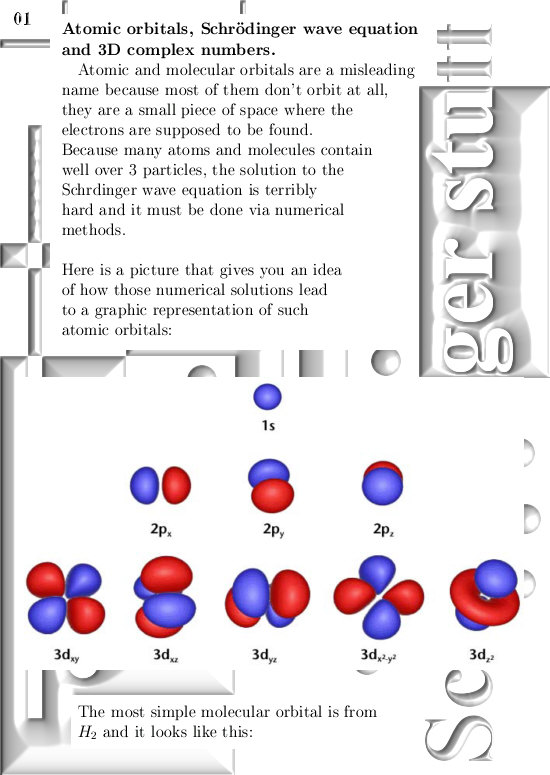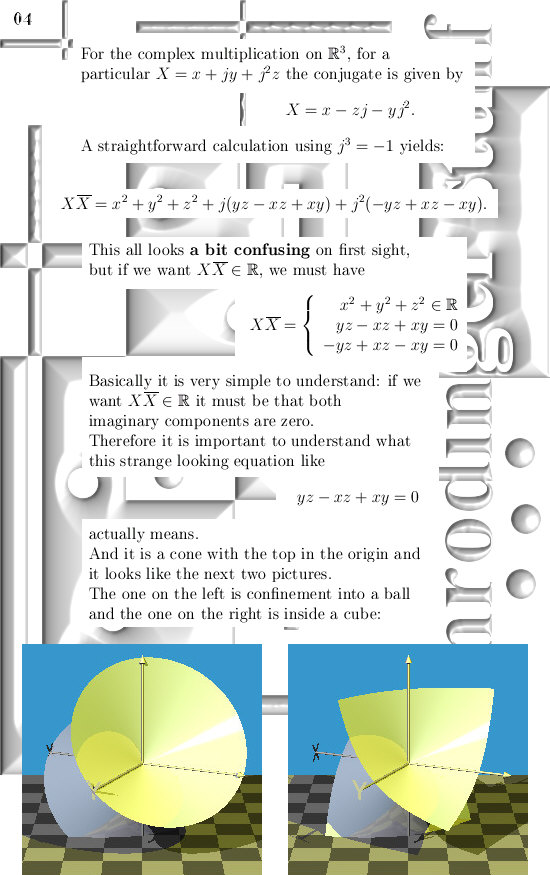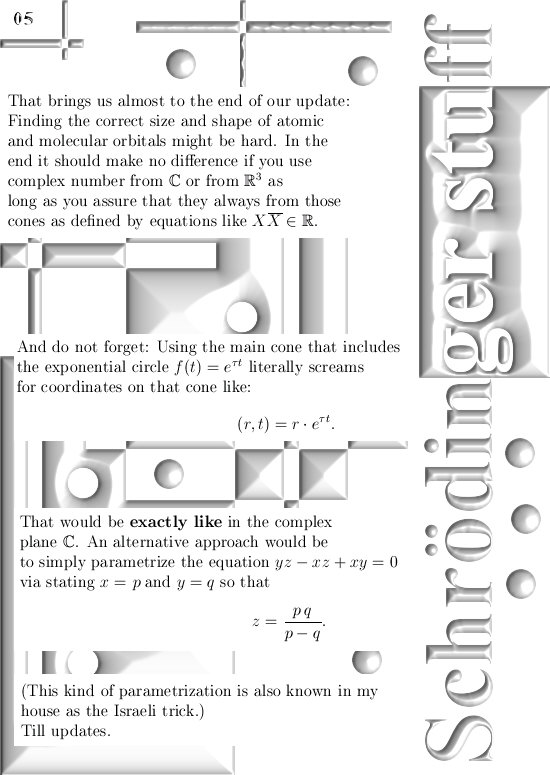Well I am happy this strange problem of invisible pictures has been solved. Till updates.

# Seven properties of the number alpha.

The number alpha is one of my best finds in the field of mathematics. In all kinds of strange ways it connects very different parts of math to one another, for example when it comes to partial differential equations the number alpha plays a crucial role in transforming this of a pile of difficult stuff into something that lives in only one dimension.

You can also use the number alpha for perpendicular projections, you can use it for this and you can use it for that.

__________

Now in the previous post I told you I would write out some of the elementary properties of the number alpha, but when I finished it the thing was about 5 A4 size pages long and that would be about 10 pictures on this new website.

That would be a bit too long and also I had written nothing in the page for 2016 on the other website. So I decided to hang those five A4 pages in the old website and you get a few teaser pictures on this new website.

Here are the three teaser pictures, click on any to land on the alpha update: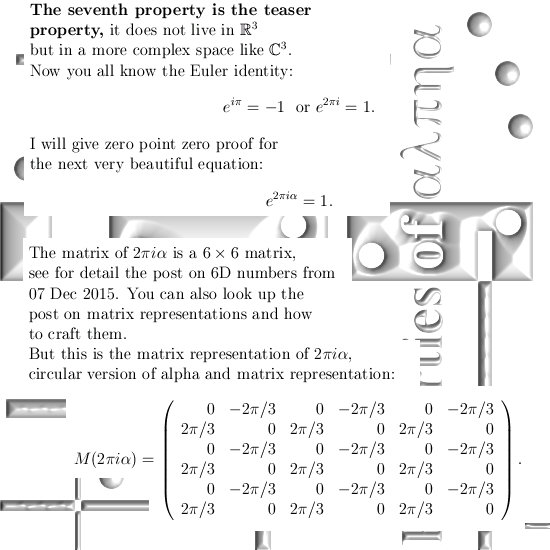The applet I used is a very helpfull tool, you can find it here:

Ok, that was it. Till updates and do not forget to floss your brain a bit every now and then…

# Calculating the Laplacian using the Cauchy-Riemann equations.

Without doubt the Laplacian is a very important differential operator. It plays a major role in for example the classical wave equation and also the Schrödinger wave equation from quantum mechanics.

Now scroll a bit back until you find the post on the Cauchy-Riemann equations, at the end I used the phrase ‘Cauchy-Riemann equations chain rule style’ and this is how we can crack in a very easy way how the Laplacian operates on functions that obey the CR equations on 3D complex numbers.

I have hundreds and hundreds of pages of math stuff on the 3D complex number system and very often I use the number alpha. This number alpha is so important, not only in 3D, that it is worth to post a few posts on them.

For the time being, I just conducted a simple Google search on the phrase ‘3d complex numbers’ in the search detail for pictures. And every time this old teaser picture from the other website pops up: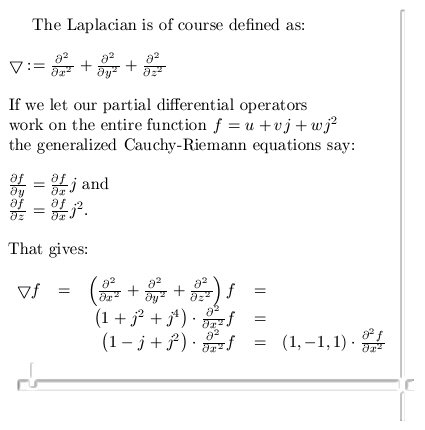At the end you see that (1, -1, 1), well that is three times alpha.

It is a nice exercise to prove that the square of alpha equals alpha.
So alpha is in the same category as for example numbers like 0 and 1 because if you square those you also get the original number back in return.

After all one squared equals one and zero squared equals zero.

End of this update, till updates.